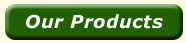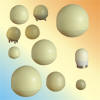Monostatic radar reflectors are used with radar systems in which the transmit antenna and the receive antenna are the same or located at the same place. Our radar reflectors are  Monostatic Radar Reflector .Luneberg Lens 2.7 inches Part No Diameter (inches) RCS (sq meter) Frequency (GHz) View Angle Polarization Weight (KG) Type 9991 2.7 0.25 10 80 Rectilinear 0.08 M

 Luneberg Lens 4.0 inches Part No Diameter (inches) RCS (sq meter) Frequency (GHz) View Angle Polarization Weight (KG) Type 9910 4.0 0.7 10 90 Rectilinear 0.18 M

 Luneberg Lens 5.3 inches Part No Diameter (inches) RCS (sq meter) Frequency (GHz) View Angle Polarization Weight (KG) Type 9010 5.3 2.0 10 90-100 Rectilinear 0.65 M

 Luneberg Lens 5.3 inches Part No Diameter (inches) RCS (sq meter) Frequency (GHz) View Angle Polarization Weight (KG) Type 9100 5.3 3.5 10 90-100 Rectilinear 0.62 M

 Luneberg Lens 6 inches Part No Diameter (inches) RCS (sq meter) Frequency (GHz) View Angle Polarization Weight (KG) Type 9019 6.0 3.0 10 110 Rectilinear 1.0 M

 Luneberg Lens 6.1 inches Part No Diameter (inches) RCS (sq meter) Frequency (GHz) View Angle Polarization Weight (KG) Type 9091 6.1 4.0 10 90-110 Rectilinear 1.05 M

 Luneberg Lens 7.0 inches Part No Diameter (inches) RCS (sq meter) Frequency (GHz) View Angle Polarization Weight (KG) Type 9001 7.0 5.5 10 100 Rectilinear 1.5 M

 Luneberg Lens 8.5 inches Part No Diameter (inches) RCS (sq meter) Frequency (GHz) View Angle Polarization Weight (KG) Type 9919 8.5 15 10 100 Rectilinear 2.5 M

 Luneberg Lens 9.0 inches Part No Diameter (inches) RCS (sq meter) Frequency (GHz) View Angle Polarization Weight (KG) Type 9901 9.0 10 10 100 Rectilinear 3.6 M